Technology
General

## Migrating Drupal 7 Forums into an Adxstudio Portal in Microsoft Dynamics – Part 2

Continuing on from Part 1 of this post, we’re going to create the PHP scripts that will pull all of your threads, posts and comments into its own XML files. These files will then be imported into DCRM through the Import interface.

## Excel XML Structure

The XML files have to be recognized as an Excel XML (XML Spreadsheet 2003) document. Otherwise, it just won’t work. So, we’ll go ahead and create this using some straightforward PHP. I’ve broken this up into multiple files, but you are able to do this in single files for each type (thread, post, comment, update). It’s set up so that once you visit the page through your browser, it automatically starts the download of the XML document.

This header is generic and you’ll be using it for all 4 scripts.

```<?php
\$xml_content = '<?xml version="1.0" encoding="utf-8"?>'."\xA";
\$xml_content .= 'xmlns:o="urn:schemas-microsoft-com:office:office"'."\xA";
\$xml_content .= 'xmlns:x="urn:schemas-microsoft-com:office:excel"'."\xA";
\$xml_content .= 'xmlns:html="http://www.w3.org/TR/REC-html40">'."\xA";
\$xml_content .= '<DocumentProperties xmlns="urn:schemas-microsoft-com:office:office">'."\xA";
\$xml_content .= '<Author>'.\$author.'</Author>'."\xA";
\$xml_content .= '<LastAuthor>'.\$author.'</LastAuthor>'."\xA";
\$xml_content .= '<Created>'.date('Y-m-d').'T'.date('H:i:s').'Z</Created>'."\xA";
\$xml_content .= '<Company>'.\$company.'</Company>'."\xA";
\$xml_content .= '<Version>14.0</Version>'."\xA";
\$xml_content .= '</DocumentProperties>'."\xA";
\$xml_content .= '<OfficeDocumentSettings xmlns="urn:schemas-microsoft-com:office:office">'."\xA";
\$xml_content .= '<AllowPNG/>'."\xA";
\$xml_content .= '</OfficeDocumentSettings>'."\xA";
\$xml_content .= '<ExcelWorkbook xmlns="urn:schemas-microsoft-com:office:excel">'."\xA";
\$xml_content .= '<WindowHeight>15260</WindowHeight>'."\xA";
\$xml_content .= '<WindowWidth>25600</WindowWidth>'."\xA";
\$xml_content .= '<WindowTopX>14120</WindowTopX>'."\xA";
\$xml_content .= '<WindowTopY>2480</WindowTopY>'."\xA";
\$xml_content .= '<ProtectStructure>False</ProtectStructure>'."\xA";
\$xml_content .= '<ProtectWindows>False</ProtectWindows>'."\xA";
\$xml_content .= '</ExcelWorkbook>'."\xA";
\$xml_content .= '<Styles>'."\xA";
\$xml_content .= '<Style ss:ID="Default" ss:Name="Normal">'."\xA";
\$xml_content .= '<Alignment ss:Vertical="Bottom"/>'."\xA";
\$xml_content .= '<Borders/>'."\xA";
\$xml_content .= '<Font ss:FontName="Calibri" x:Family="Swiss" ss:Size="12" ss:Color="#000000"/>'."\xA";
\$xml_content .= '<Interior/>'."\xA";
\$xml_content .= '<NumberFormat/>'."\xA";
\$xml_content .= '<Protection/>'."\xA";
\$xml_content .= '</Style>'."\xA";
\$xml_content .= '</Styles>'."\xA";
\$xml_content .= '<Worksheet ss:Name="Sheet1">'."\xA";
?>
```

#### The Footer – footer.php

Similar to the header, the footer is also generic. However, we are inserting the total number of records we obtained from the SQL query.

```<?php
\$xml_content .= '<WorksheetOptions xmlns="urn:schemas-microsoft-com:office:excel">'."\xA";
\$xml_content .= '<Print>'."\xA";
\$xml_content .= '<ValidPrinterInfo/>'."\xA";
\$xml_content .= '<HorizontalResolution>-4</HorizontalResolution>'."\xA";
\$xml_content .= '<VerticalResolution>-4</VerticalResolution>'."\xA";
\$xml_content .= '</Print>'."\xA";
\$xml_content .= '<PageLayoutZoom>0</PageLayoutZoom>'."\xA";
\$xml_content .= '<Selected/>'."\xA";
\$xml_content .= '<Panes>'."\xA";
\$xml_content .= '<Pane>'."\xA";
\$xml_content .= '<Number>'.\$num_rows.'</Number>'."\xA";
\$xml_content .= '<ActiveRow>2</ActiveRow>'."\xA";
\$xml_content .= '<ActiveCol>1</ActiveCol>'."\xA";
\$xml_content .= '</Pane>'."\xA";
\$xml_content .= '</Panes>'."\xA";
\$xml_content .= '<ProtectObjects>False</ProtectObjects>'."\xA";
\$xml_content .= '<ProtectScenarios>False</ProtectScenarios>'."\xA";
\$xml_content .= '</WorksheetOptions>'."\xA";
\$xml_content .= '</Worksheet>'."\xA";
\$xml_content .= '</Workbook>'."\xA";
echo \$xml_content;
?>
```

## The XML Scripts

We’re going to dive in a little deeper into the XML scripts we talked about in Part 1. We went over the initial layout and what data each one will have. Now, we’re going to build out each script along with its respective data.

In this file, we’re running a query to pull all of the forum threads from the database. It’s grabbing the name of the thread and the last post date. We’re manually specifying the Forum that the thread belongs to, as well as the type of thread.

```<?php
\$xml_content .= '<Table ss:ExpandedColumnCount="4" ss:ExpandedRowCount="1" x:FullColumns="1" x:FullRows="1" ss:DefaultColumnWidth="65" ss:DefaultRowHeight="15">'."\xA";
\$xml_content .= '<Row>'."\xA";
\$xml_content .= '<Cell><Data ss:Type="String">Forum</Data></Cell>'."\xA";
\$xml_content .= '<Cell><Data ss:Type="String">Last Post Date</Data></Cell>'."\xA";
\$xml_content .= '<Cell><Data ss:Type="String">Name</Data></Cell>'."\xA";
\$xml_content .= '<Cell><Data ss:Type="String">Type</Data></Cell>'."\xA";
\$xml_content .= '</Row>'."\xA";

//Create Rows of Records
\$result = mysql_query(\$query);
\$num_rows = mysql_num_rows(\$result);
while (\$row = mysql_fetch_object(\$result)) {
\$xml_content .= '<Row>'."\xA";
\$xml_content .= '<Cell><Data ss:Type="String">NAME OF YOUR FORUM IN DCRM</Data></Cell>'."\xA";
\$xml_content .= '<Cell><Data ss:Type="String">'.\$row->Last_Post_Date.'</Data></Cell>'."\xA";
\$xml_content .= '<Cell><Data ss:Type="String">'."\xA";
\$xml_content .= '<![CDATA['."\xA";
\$xml_content .= ']]>'."\xA";
\$xml_content .= '</Data></Cell>'."\xA";
\$xml_content .= '<Cell><Data ss:Type="String">NAME OF FORUM THREAD TYPE</Data></Cell>'."\xA";
\$xml_content .= '</Row>'."\xA";
}
mysql_close();

//End the Table
\$xml_content .= '</Table>'."\xA";
?>
```

#### XML Script 2 (Forum Posts) – adx_forum_posts.php

With out threads set up, we now need the forum posts. We’ll running a query to pull all of the forum posts from the database. To be more specific, we’re actually pulling in the initial posts that were created for the thread. The way this works in Adxstudio is that when you are creating a new thread, it creates the thread record first and then adds the post (which is no different than a comment) to that thread.

So, we’re naming our first post the same as the Thread’s name. You’ll also notice that for the Author, we’re using the email address. This ensures that the post is assigned correctly (make sure the user already exists as a Contact), otherwise the import will fail if you happen to have two people with the exact same name.

```<?php
\$xml_content .= '<Table ss:ExpandedColumnCount="5" ss:ExpandedRowCount="1" x:FullColumns="1" x:FullRows="1" ss:DefaultColumnWidth="65" ss:DefaultRowHeight="15">'."\xA";
\$xml_content .= '<Row>'."\xA";
\$xml_content .= '<Cell><Data ss:Type="String">Author</Data></Cell>'."\xA";
\$xml_content .= '<Cell><Data ss:Type="String">Date</Data></Cell>'."\xA";
\$xml_content .= '<Cell><Data ss:Type="String">Name</Data></Cell>'."\xA";
\$xml_content .= '<Cell><Data ss:Type="String">Content</Data></Cell>'."\xA";
\$xml_content .= '</Row>'."\xA";

//Create Rows of Records
\$result = mysql_query(\$query);
\$num_rows = mysql_num_rows(\$result);
while (\$row = mysql_fetch_object(\$result)) {
\$xml_content .= '<Row>'."\xA";
\$xml_content .= '<Cell><Data ss:Type="String">'.\$row->Last_Post_Date.'</Data></Cell>'."\xA";
\$xml_content .= '<Cell><Data ss:Type="String">'."\xA";
\$xml_content .= '<![CDATA['."\xA";
\$xml_content .= ']]>'."\xA";
\$xml_content .= '</Data></Cell>'."\xA";
\$xml_content .= '<Cell><Data ss:Type="String">'."\xA";
\$xml_content .= '<![CDATA['."\xA";
\$xml_content .= ']]>'."\xA";
\$xml_content .= '</Data></Cell>'."\xA";
\$xml_content .= '<Cell><Data ss:Type="String">'."\xA";
\$xml_content .= '<![CDATA['."\xA";
\$xml_content .= nl2p(\$row->Content, false, true)."\xA";
\$xml_content .= ']]>'."\xA";
\$xml_content .= '</Data></Cell>'."\xA";
\$xml_content .= '</Row>'."\xA";
}
mysql_close();

//End the Table
\$xml_content .= '</Table>'."\xA";
?>
```

What’s a forum post without comments? Now, we’ll run another query to pull all of the forum comments from the database. In essence, this isn’t any different than the initial post. The main difference is time (since the comment came after the first post), and that we’ve given it a unique string as a Name.

```<?php
\$xml_content .= '<Table ss:ExpandedColumnCount="5" ss:ExpandedRowCount="1" x:FullColumns="1" x:FullRows="1" ss:DefaultColumnWidth="65" ss:DefaultRowHeight="15">'."\xA";
\$xml_content .= '<Row>'."\xA";
\$xml_content .= '<Cell><Data ss:Type="String">Author</Data></Cell>'."\xA";
\$xml_content .= '<Cell><Data ss:Type="String">Date</Data></Cell>'."\xA";
\$xml_content .= '<Cell><Data ss:Type="String">Name</Data></Cell>'."\xA";
\$xml_content .= '<Cell><Data ss:Type="String">Content</Data></Cell>'."\xA";
\$xml_content .= '</Row>'."\xA";

//Create Rows of Records
\$query = 'SELECT Email_Address, Last_Post_Date, Thread_Name, unique_string, Content FROM forum_posts WHERE uid!=0;';
\$result = mysql_query(\$query);
\$num_rows = mysql_num_rows(\$result);
while (\$row = mysql_fetch_object(\$result)) {
\$xml_content .= '<Row>'."\xA";
\$xml_content .= '<Cell><Data ss:Type="String">'.\$row->Last_Post_Date.'</Data></Cell>'."\xA";
\$xml_content .= '<Cell><Data ss:Type="String">'."\xA";
\$xml_content .= '<![CDATA['."\xA";
\$xml_content .= ']]>'."\xA";
\$xml_content .= '</Data></Cell>'."\xA";
\$xml_content .= '<Cell><Data ss:Type="String">'."\xA";
\$xml_content .= '<![CDATA['."\xA";
\$xml_content .= \$row->unique_string."\xA";
\$xml_content .= ']]>'."\xA";
\$xml_content .= '</Data></Cell>'."\xA";
\$xml_content .= '<Cell><Data ss:Type="String">'."\xA";
\$xml_content .= '<![CDATA['."\xA";
\$xml_content .= nl2p(\$row->Content, false, true)."\xA";
\$xml_content .= ']]>'."\xA";
\$xml_content .= '</Data></Cell>'."\xA";
\$xml_content .= '</Row>'."\xA";
}
mysql_close();

//End the Table
\$xml_content .= '</Table>'."\xA";
?>
```

In this file, we’re running a query to pull all of the forum posts along with the first post, last post and post counts. Essentially, we’re going to “update” the threads with this information. It couldn’t be done in the beginning because the First and Last Post ID’s don’t exist – so this part would fail.

```<?php
\$xml_content .= '<Table ss:ExpandedColumnCount="5" ss:ExpandedRowCount="1" x:FullColumns="1" x:FullRows="1" ss:DefaultColumnWidth="65" ss:DefaultRowHeight="15">'."\xA";
\$xml_content .= '<Row>'."\xA";
\$xml_content .= '<Cell><Data ss:Type="String">Name</Data></Cell>'."\xA";
\$xml_content .= '<Cell><Data ss:Type="String">First Post</Data></Cell>'."\xA";
\$xml_content .= '<Cell><Data ss:Type="String">Last Post</Data></Cell>'."\xA";
\$xml_content .= '<Cell><Data ss:Type="String">Post Count</Data></Cell>'."\xA";
\$xml_content .= '</Row>'."\xA";

//Create Rows of Records
\$result = mysql_query(\$query);
\$num_rows = mysql_num_rows(\$result);
while (\$row = mysql_fetch_object(\$result)) {
\$xml_content .= '<Row>'."\xA";

\$xml_content .= '<Cell><Data ss:Type="String">'."\xA";
\$xml_content .= '<![CDATA['."\xA";
\$xml_content .= ']]>'."\xA";
\$xml_content .= '</Data></Cell>'."\xA";

\$xml_content .= '<Cell><Data ss:Type="String">'."\xA";
\$xml_content .= '<![CDATA['."\xA";
\$xml_content .= ']]>'."\xA";
\$xml_content .= '</Data></Cell>'."\xA";

\$xml_content .= '<Cell><Data ss:Type="String">'."\xA";
\$xml_content .= '<![CDATA['."\xA";
\$xml_content .= \$row->First_Post_Id."\xA";
\$xml_content .= ']]>'."\xA";
\$xml_content .= '</Data></Cell>'."\xA";

\$xml_content .= '<Cell><Data ss:Type="String">'.\$row->Last_Post_Id.'</Data></Cell>'."\xA";
\$xml_content .= '<Cell><Data ss:Type="String">'.\$row->Post_Count.'</Data></Cell>'."\xA";
\$xml_content .= '</Row>'."\xA";
}
mysql_close();

//End the Table
\$xml_content .= '</Table>'."\xA";
?>
```

Note: This is the last script we’re going to import. However, a workflow has to be set up. This will be covered in Part 3.

## Bringing it all together

Now that we have our header, footer and 4 different files, we just need to write a PHP script that will call each of the 4 scripts individually. I’ll use the threads as an example.

The only thing to keep note of here is the file. Make sure you set the right name for your filename. It makes it easier to know what each XML file is later. You’ll notice that we added time to the filename. It keeps the filename unique in the event you were to download this more than once.

```<?php
\$current_time = date('h_i_s');

//Changes new lines to paragraphs
//Found on Stack Overflow - http://stackoverflow.com/questions/7409512/new-line-to-paragraph-function
function nl2p(\$string, \$line_breaks = true, \$xml = true) {

\$string = str_replace(array('<p>', '</p>', '<br>', '<br />'), '', \$string);

// It is conceivable that people might still want single line-breaks
// without breaking into a new paragraph.
if (\$line_breaks == true)
return '<p>'.preg_replace(array("/([\n]{2,})/i", "/([^>])\n([^<])/i"), array("</p>\n<p>", '\$1<br'.(\$xml == true ? ' /' : '').'>\$2'), trim(\$string)).'</p>';
else
return '<p>'.preg_replace(
array("/([\n]{2,})/i", "/([\r\n]{3,})/i","/([^>])\n([^<])/i"),
array("</p>\n<p>", "</p>\n<p>", '\$1<br'.(\$xml == true ? ' /' : '').'>\$2'),

trim(\$string)).'</p>';
}

require_once 'footer.php';
?>
```

## Running the Import

We’ll start by going to the Forums section in DCRM and then clicking on Import Data.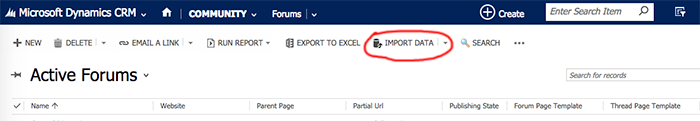This should bring up an Upload dialogue window. Select your XML script, e.g. forum_threads, and start the upload. Once the upload completes, you should see the following dialogue window.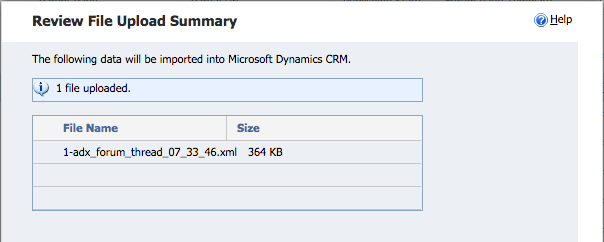Once you press Next, you’ll get the following dialogue window asking you to Select a Data Map. If this is your first import, stick with the default and choose Automatic Mapping. You’ll get the option to set the mapping of the data yourself. Once you’re complete, you can save this Data Map for future use so you don’t have to go through the mapping process again.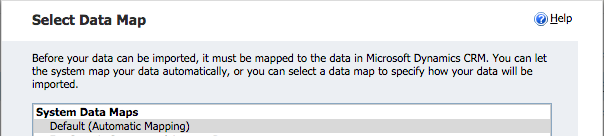The next dialogue window will more than likely tell you it couldn’t determine what CRM Record type you’re going to be importing the forum threads to.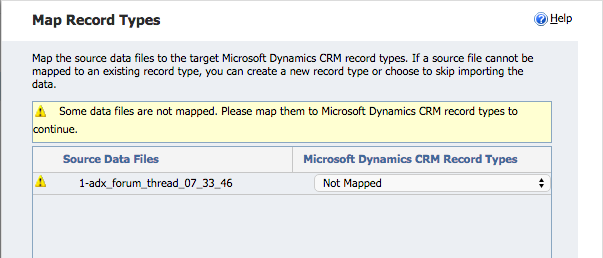From the drop down list, choose Forum Thread and proceed to the next dialogue which should have your columns in your XML file mapped to the field in the DCRM record.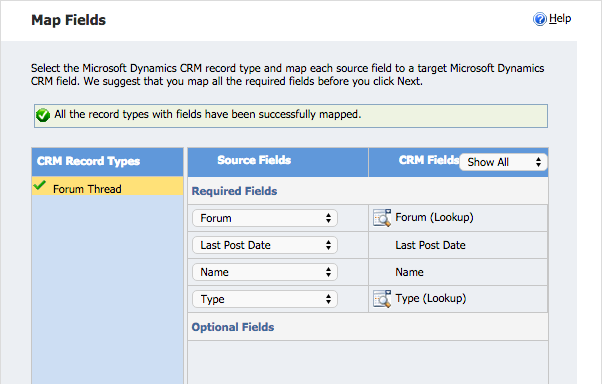You’re now done with mapping the fields in the DCRM record type and can start importing your data. Make sure to go through the same steps with XML Scripts 1, 2 and 3. We’re skipping 4 as that involves a few extra steps.

#### Modifying the Mapping of the Author

In the XML Scripts for 2 and 3 (threads and posts), we’ve set up our script to use an email address since this is more unique than a full name. If you stick to using a full name and you have two contacts by the name of “John Doe”, the import script will fail because it won’t know who to associate the forum post to.

In this case, you simply change the mapping of the Author to the email address in the Contact record.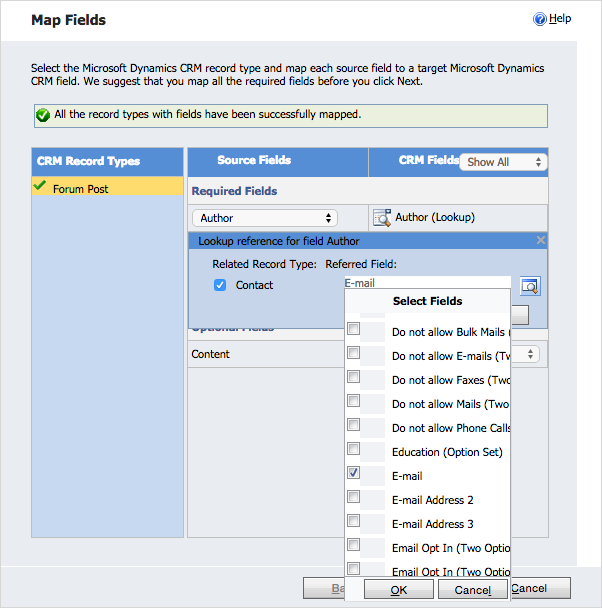## What to Expect in Part 3

In Part 3, we’ll go over creating Entities and Workflows within DCRM so that we could update the values on the Forum Thread (XML Script 4). This part is essential as it ties in the post with it’s comments.